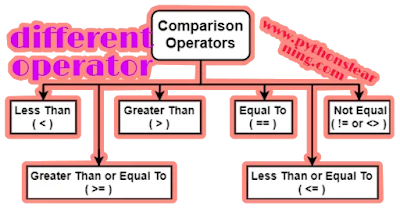# 1. Python Comparison Operators

Welcome everyone,Today we will focus our Topic Python Comparison Operators. These is relational operators in Python.
also we will learn different types of Comparison Operators in Python:

• less than
• greater than
• less than
•  greater than or equal to,and not equal to with simple example
So, let’s start:greater than or equal to python operators

## Types of Python Comparison Operators

A python relational operator is also called comparison operator in python, It’s work on very simple basic tech. it compare the value of two operand and return True or False value based on whether it met the conditions . Also see : Attributes in python 3

### 1. Python Less Than (<) Operator

The first operator in python is comparison operator and it is  Denoted by the symbol <,
on which logic less than operator works:
It is simply check if the left value is lesser than the right value then it check condition we see some example:
15<11
Output:
False
11 is less than 15 so, it returns False.
2<20
Output:
True
11 is less than 15 so, it returns True.
With these operator Float values is also be evaluated .
3.2<4.4
Output:
True
As similar like float you can try this operator with string value also.
‘X'<‘Z’
Output:
True
‘Z'<‘X’
Output:
False
we can also compare tuples with the help of  this operators.
(1,2,3,4)<(1,2,3,4,5)
Output:
True
(1,2,3,4)<(1,2,3,4)
Output:
True
It compare list by index by index.
[1,2,3,4,5]<[1,2,3,4,5]
Output:
True
[5,9,1]<[5,8,8]
Output:
False
Drawback is This does not work on dictionaries.

## 2.Python Greater Than (>) Operator

Let start to see the Python greater than operator(>). It is denoted by the ‘>’ symbols
on which logic less than operator works:
It observe whether the value on the left side is greater than the right side and display result.
9>5
Output:
True
Since 9 is greater than 5.
‘python’>’PYTHON’
Output:
True
In the case of string value,python compare the ASCII  character values. Here, ‘python’ ASCII value is smaller and ‘PYTHON’ ASCII value is greater.

### 3.Less Than or Equal To (<=) python

The less than or equal to python operator can be denoted by (<=) this symbol.
on which logic less than operator works:
It get output True only if the values on the left is either less than or equal to that on the right of the operators.
>>> x=4
>>> x<=x*2
Output: True
Another example:
10<=15
Output:
True
15<=10
Output:
False
operator 15<10 would give us false output.

#### 4. Greater than or equal to python(>=)

The greater than or equal to python operator is just like less than or equal to python operator
on which logic less than operator works:
It observe that the left side values should be greater than or equal to the right sides of value.
15>=10
Output:
True
34>=35
Output:
False

### 5.Equal To (==) Python Operator:

on which logic less than operator works:
The equal to python operator will return True when both of the value on either side of the operator are must be equal. You can also compare the integers, float,strings value.
15==15
Output:
True
2.0==2
Output:
True
13==13.4
Output:
False
Here, 13 is an integer value and 13.5 is float,hence they are not equal.
1. “pythonslearning” == “pythonslearning”
Output:
True
You can also compare both lists and sets.
{1,2,3,4,5}=={3,2,1,1}
Output:
False

### 6. Not equal to (!=) python operator:

The the equal to operator is opposite to not equal to operator (!=) .
on which logic less than operator works:
It returns true value when on either side are unequal to each other respectively.
“50”!=50
Output:
True
1. “Pythonslearning” != “Pythonslearning”
Output:
False

## Summary :

In this post we saw the six different comparison operators of Python named as:
• less than
• greater than
• less than
•  greater than or equal to,and not equal to with simple example

### Must Read : PYTHON INTERVIEW QUESTIONS for beginner-experienced professionals asked in MNC

Some FAQ about  Python Comparison Operators :

1. which python basic operators ?
• Arithmetic Operators
• Comparison (Relational) Operators
• Assignment Operators
• Logical Operators
• Bitwise Operators
• Membership Operators
• Identity Operators
2.  which is  Python Comparison Operators  ?
• less than
• greater than
• less than
•  greater than or equal to,and not equal to with simple example
If you want to learn python course free then click on python tutorials point and if you like post then please share this post.
If Any doubts or problem in this section till now?  then Mention in the comment section we will solve it.

#### Also see : Machine Learning Interview questions for TCS and Wipro

Tags: In this article we covered Python Comparison Operators,greater than or equal to python operators,python basic operators point.
BEST OF LUCK!!!

### sachin PagarI am Mr. Sachin pagar Embedded system engineer, the founder of Pythonslearning, a Passionate Educational Blogger and Author, who love to share the informative content on educational resources.

### sachin Pagar

I am Mr. Sachin pagar Embedded system engineer, the founder of Pythonslearning, a Passionate Educational Blogger and Author, who love to share the informative content on educational resources.